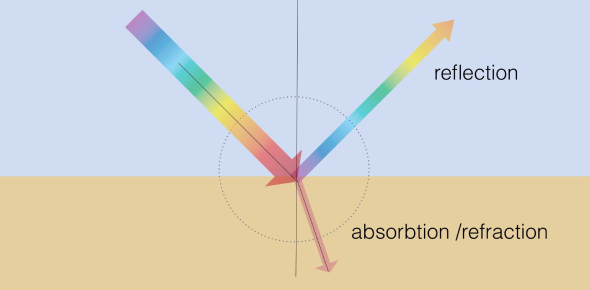# Light Quiz: Reflection And Refraction! Exam

15 Questions | Attempts: 7202Settings• 1.
An object is placed 20 cm in front of a plane mirror. The mirror is moved 2 cm towards the object. The distance between the positions of the original and final images seen in the mirror is: An object is placed 20 cm in front of a plane mirror. The mirror is moved 2 cm towards the object. The distance between the positions of the original and final images seen in the mirror is:
• A.

2 cm

• B.

4 cm

• C.

10 cm

• D.

22 cm

• 2.
A ray of light strikes a plane mirror PQ at an angle of incidence of 30o, is reflected from the plane mirror, and then strikes a second plane mirror QR placed at right angles to the first mirror. The angle of reflection at the second mirror is:
• A.

30o

• B.

45o

• C.

60o

• D.

90o

• 3.
An object is 100 mm in front of a concave mirror which produces an upright image (erect image). The radius of curvature of the mirror is:
• A.

Less than 100 mm

• B.

Between 100 mm and 200 mm

• C.

Exactly 200 mm

• D.

More than 200 mm

• 4.
Which position of the object will produce a magnified virtual image, if a concave mirror of focal length 15 cm is being used?
• A.

10 cm

• B.

20 cm

• C.

30 cm

• D.

35 cm

• 5.
A concave mirror produces a magnification of +4. The object is placed:
• A.

At the focus

• B.

Between focus and center of curvature

• C.

Between focus and pole

• D.

Beyond the center of curvature

• 6.
A lens of focal length 12 cm forms an erect image, three times the size of the object. The distance between the object and image is:
• A.

8 cm

• B.

16 cm

• C.

24 cm

• D.

36 cm

• 7.
Two big mirrors A and B are fitted side by side on a wall. A man is standing at such a distance from the wall that he can see the erect image of his face in both the mirrors. When the man starts walking towards the mirrors, he finds that the size of his face in mirror A goes on increasing but that in mirror B remains the same:
• A.

Mirror A is concave and mirror B is convex

• B.

Mirror A is plane and mirror B is concave

• C.

Mirror A is concave and mirror B is plane

• D.

Mirror A is convex and mirror B is concave

• 8.
If an object is placed 21 cm from a converging lens, the image formed is slightly smaller than the object. If the object is p[laced 19 cm from the lens, the image formed is slightly larger than the object. The approximate focal length of the lens is:
• A.

20 cm

• B.

18 cm

• C.

10 cm

• D.

5 cm

• 9.
A ray of light traveling in waterfalls at right angles to the boundary of a parallel-sided glass block. The ray of light:
• A.

Is refracted towards the normal

• B.

Is refracted away from the normal

• C.

Is reflected along the same path

• D.

Does not get refracted

• 10.
A ray of light passes from a medium X to another medium Y. No refraction of light occurs if the ray of light hits the boundary of medium Y at an angle of:
• A.

120o

• B.

90o

• C.

45o

• D.

0o

• 11.
A student does the experiment on tracing the path of a ray of light passing through a rectangular glass slab for different angles of incidence. He can get a correct measure of the angle of incidence and the angle of emergence by following the labeling indicated in the figure:
• A.

A

• B.

B

• C.

C

• D.

D

• 12.
While performing an experiment on the determination of the focal length of a convex lens, four students obtained the image of the same distant tree on the screen as follows: Which diagram shows the formation of the image correctly?
• A.

A

• B.

B

• C.

C

• D.

D

• 13.
A student traces the path of a ray of light passing through a rectangular slab. For measuring the angle of incidence, he must position the protractor in thye manner shown in the figure:
• A.

A

• B.

B

• C.

C

• D.

D

• 14.
Four students A, B, C, and D, experimented to determine the focal length of a concave mirror by obtaining a distant tree's image on a screen. They measured the distances between the screen and the mirror as shown in the diagrams given below: The student will obtain the correct value for focal length:
• A.

A

• B.

B

• C.

C

• D.

D

• 15.
A student traces the path of a ray of light passing through a rectangular slab for three different values of angle of incidence (∠i ) namely 30o, 45o, and 60o. He extends the direction of the incident ray by a dotted line and measures the perpendicular distance ‘l’ between the extended incident ray and the emergent ray. He will observe that:
• A.

‘l’ keeps on increasing with increase in angle of incidence

• B.

‘l’ keeps on decreasing with increase in angle of incidence

• C.

‘l’ remains the same for all three angles of incidence

• D.

‘l’ is the maximum for ∠i = 45o and is less than this value for ∠i = 30o and ∠i = 60o

## Related TopicsBack to top
×

Wait!
Here's an interesting quiz for you.